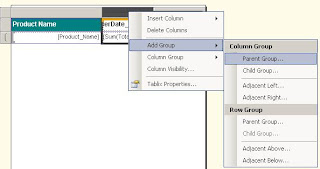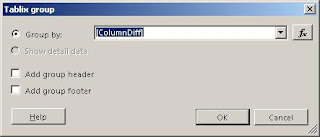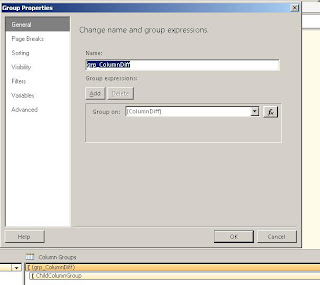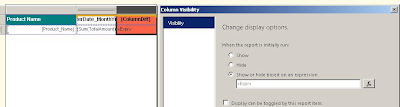## Monday, 26 November 2012

### Format DateTime into Quarter

Format DateTime into Quarter
Most of the time, we format DateTime value in various formats like day, month year etc. If we have to format a DateTime value into Quarter, we can use following expression syntax
=Format(DatePart(DateInterval.Quarter, <Date Value>))

For instance,
=Format(DatePart(DateInterval.Quarter, cdate("11/26/2012"))) will result 4.
AND
="Q"+Format(DatePart(DateInterval.Quarter, cdate("11/26/2012")))+","+cdate("11/26/2012").Year().ToString() will result Q4,2012
AND in following expression, we are going backward to 4 quarters
Will result Q4,2011

## Sunday, 25 November 2012

### Calculate Column difference in Matrix

Sometimes we fall into a situation where we need to calculate columns difference in Matrix. Something likes image1﻿﻿﻿Image1
﻿﻿Let’s go through with following steps to achieve this output in Matrix. I believe that you already have created required column and row group in your matrix. The following steps are additional work to achieve the column difference in the matrix.
Step 1. Create a calculated column in the dataset named as ColumnDiff and assign this expression under Field SourceImage2
Refer image2 regarding this step.

Step 2. Now select the matrix and create a new Parent Group under your Column GroupsImage3
Refer image3 regarding this step.

Choose [ColumnDiff] field as a Group by expression and click ok button.Image4
Refer image4 regarding this step.

Now click on newly created column group and give an approprite name as grp_ColumnDiff. Because we will use this group name in a expression in next step.Image5
Refer image5 regarding this step.

Step 3.  Now use following expression in your child group row & [ColumnDiff] column cell.
=SUM(Fields!TotalAmount.Value)-Previous(SUM(Fields!TotalAmount.Value),"grp_ColumnDiff")
Refer image6 regarding this step.Image6

Let’s discuss the above expression.
SUM(Fields!TotalAmount.Value) will give the total value for the current column group and Previous(SUM(Fields!TotalAmount.Value),"grp_ColumnDiff") will give the total value of the previous column group corrosponding to grp_ColumnDiff column group

Step 4. Now set the following column visibilty expression for the last column that says [ColumnDiff]
=CBOOL(Cdate(Fields!OrderDate.Value).Month mod 2)
Refer image7 regarding this step.Image7

This expression will return True for ODD month number and False for even month number.

You need to only change the report connection to appropriate connection string to execute this report at your own enviornment. This sample is designed in SSRS2008R2 version. So lower version of SSRS might give some design error.

## Friday, 23 November 2012

### ProperCase in SSRS

Probably, we all have used UpperCase/LowerCase text formatting by using UCase/LCase method in SSRS. But if we have to use ProperCase then SSRS does not give any direct method for this.
To use ProperCase in SSRS, we need to use following expression syntax:
=Microsoft.VisualBasic.StrConv(<Text Expression>,Microsoft.VisualBasic.VbStrConv.ProperCase)

For example, following expression
=Microsoft.VisualBasic.StrConv("this is a propercase example.",Microsoft.VisualBasic.VbStrConv.ProperCase)
Will give the output as
This Is A Propercase Example.

## Thursday, 15 November 2012

### Read wild card character as Charater

Requirement :- Let's have a sample data as below and you need to search the data started with %, _ in your table
Id
Item
1    _Computer
2

%Computer
3
%Desk
4
%pen
_
pen
Create table #test (ID int , Item varchar(50))
insert into #test values (1,'_Computer')
insert into #test values (2,'%Computer')
insert into #test values (3,'%Desk')
insert into #test values (4,'%Pen')
insert into #test values (5,'_Pen')

Solution :- Just put your wild card character in open and closed square brackets
Example :-
Select * from #test where Item like '[%]%'

### Rank and Dense Rank in MDX

WITH
MEMBER [Measures].[Rank]ASRank(
[Product].[Model Name].
CurrentMember,[Product].[Model Name].[Model Name],
[Measures].[ORDER Count]
)

SET [Sorted Models]AS
ORDER
(
[Product].[Model Name].[Model Name]
,[Measures].[ORDER Count]
,
BDESC)MEMBER [Measures].[Previous Model INDEX]AS(Rank(
[Product].[Model Name].
CurrentMember,[Sorted Models]
)
-
2
)

MEMBER [Measures].[Dense Rank]AS
Case
When [Measures].[Rank] = 1
Then 1
Else(
[Sorted Models].
Item([Measures].[Previous Model INDEX]),[Measures].[Dense Rank]
)
+

Iif(
(
[Sorted Models].
Item([Measures].[Previous Model INDEX]),[Measures].[ORDER Count]
)
=
[Measures].[ORDER Count]
,0
,1
)
EndSELECT{
[Measures].[Rank],
[Measures].[Dense Rank]
}
ON 0,{
[Sorted Models]
}

## Tuesday, 13 November 2012

### How to remove all numeric/non-numeric characters from the string in SSRS

Let’s say we have a string like 1;B4Y;dce5;6fgh;This
And we are expecting an output like this BY;dce;fgh;This
To remove all the numeric characters from a string, we need to use regular expression like following:
=System.Text.RegularExpressions.Regex.Replace("1;B4Y;dce5;6fgh;This", "[0-9]", "").Trim(";")
This expression will give the output as BY;dce;fgh;This

And vise versa, if we want only numbers then we need to use regular expression like following:
=System.Text.RegularExpressions.Regex.Replace("1;B4Y;dce5;6fgh;This", "[^0-9]", "").Trim(";")
This expression will give the output as 1456